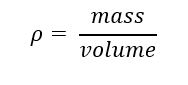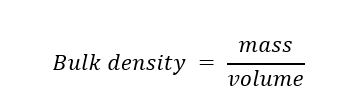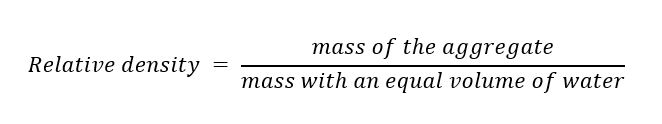# What is the Density of Aggregate in kg/m3?

## What is the Density of Aggregate in kg/m3?

Building materials must satisfy a wide variety of criteria because of the wide range of uses for structures and constructions as well as the different production processes.

Building materials may be categorised into physical and chemical properties, and one of the most important physical properties is the density of construction materials.

In this blog, we will learn about the density of aggregate in kg/m3. Aggregate provides the completed building material with volume, stability, tolerance to abrasion or corrosion, and other necessary physical attributes.

## Density

The definition of density is the mass of a substance per unit volume. The letter D can also be used to signify density instead of the symbol ρ.

The mass per unit volume of the materials utilised or in issue is the density of construction materials. The compactness of the construction material is indicated by the S.I. units of expression, which is kg/m3.

##In this equation,

ρ = the density [kg / m3]

m =the mass [kg]

V = the volume [m3]

## Aggregate

One of the crucial components of building projects is aggregate, which is combined with water and Portland cement to create the crucial component known as concrete. Concrete’s primary component is known as aggregate.

This is a collection of non-metallic minerals that can be processed and utilised quickly for building civil and road engineering since they are acquired as particles.

### Types of Aggregate

Generally speaking, there are two types of aggregates:

1. The range of fine aggregate size of particles is typically 75 micrometres to 4.75 mm. In concrete, fine aggregate fills the space or air pocket between the coarse aggregate.
2. According to IS, coarse aggregate has a particle size of more than 4.75 mm. The major component of concrete is made up of coarse aggregate in addition to sand, cement, and water.

The largest size of coarse material that may be used to make concrete is 20 mm. Construction-related aggregates must be chemically inert by nature.

### Bulk Density of Aggregate in kg/m3

The weight and mass of an aggregate required to fill a vessel with a specific unit volume are referred to as its density or unit weight.

##Volume equals one unit, hence bulk density equals mass.

The units are either kg/m3 or lb/ft3.

The volume mentioned in the above definition includes the intermolecular spaces and aggregates in between the aggregate particles, with a relative density of around 1200-1750 kg/m3, or 75-110 lb/ft3, which is the average relative density of the aggregate frequently used in normal-weight concrete.

### Standard Relative Density of Aggregate in kg/m3

The ratio of mass of an identical amount of water to a mass is known as the specific gravity or relative density of an aggregation.

##The relative density of the aggregates will be between 2.4 and 2.9, and the mass particle density of aggregate in kg/m3 will be between 2400 and 2900 kg/m3 (150 to 181 lb per ft3).

### Artificial Density of Aggregate

Broken bricks, synthetic aggregates, and blast furnace slag are examples of artificial aggregates. Brickbat, another name for broken bricks, is ideal for large-scale concrete projects. However, it is not advised to utilise them for reinforced concrete.

Blast furnace slag aggregate is a by-product of power plants made by slowly cooling the slag and then crushing it. Precast concrete products are made using these kinds of aggregate.

These have a specific gravity between 2.2 and 2.8 and a bulk density between 1120 and 1300 kg/m3.

### Conclusion: Why is Density Important in Construction?

By understanding the density of the substance, we can determine any material’s ability to sink. This implies that a substance would float on the surface if its density is lower than that of the liquid. In addition, if it is heavier than the liquid and denser, it will sink.

The density of two materials will differ, however, if their weights are equivalent for two distinct materials. Compared to materials with greater densities, those with lower densities occupy more volume.

Finding out how much material is required for a place may also be done using the density of the building materials.

### FAQs

• #### What is the density of 10mm aggregate?

The weight of one cubic metre of 10 mm coarse aggregate is approximately 1525 kg to 1575 kg, or 1.525 to 1.575 MT, depending on the density, which ranges from 1525 kg/m3 to 1575 kg/m3.

• #### What is the density of 12mm aggregate?

The density of 10mm and 12mm fine aggregate is between 1600 and 1750 kg/m3 (100 and 109 lb/ft3).

• #### What is the density of 40mm aggregate?

20mm and 40mm coarse aggregate has a density of 1200 to 1450 kg/m3 (75 to 90 lb/ft3).Channel for more information.
1.rightangledevelopers says: